Processing ......FreeComputerBooks.com Links to Free Computer, Mathematics, Technical Books all over the World

Probability Theory and Stochastic Processes with Applications
Top Free Data Science Books 🌠 - 100% Free or Open Source!
• Title Probability Theory and Stochastic Processes with Applications
• Author(s) Oliver Knill
• Publisher: Overseas Press (August 12, 2009); eBook (2009)
• Permission: The PDF is post by the author, the copyright holder.
• Hardcover 510 pages
• eBook PDF (27 MB) and DJVU (8 MB)
• Language: English
• ISBN-10/ASIN: 8189938401
• ISBN-13: 978-8189938406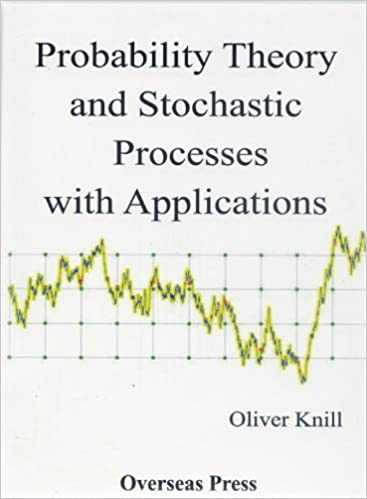Book Description

This book provides an introduction to probability theory and discrete and continuous Stochastic Processes and its applications. It has a unique approach that provides a broad and wide introduction into the fascinating area of probability theory.

The introduction summarizes the book in prose and some popular pop star problems like famous paradoxa, the mathematics starts in chapter 2. All measure theory and real analysis is covered in detail. The chapter ends with the central limit theorem and the law of the iterated log. This is all pretty standard and is the material which is covered usually in a serious probability theory course. Chapter 3 covers discrete stochastic processes and Martingales. Chapter 4 covers continuous stochastic processes like Brownian motion up to stochstic differential equations. Also chapters 3 and 4 are pretty standard material and cover what is usually offered in a second graduate course. Chapter 5 is special and makes the book unique.

There are various selected topics like Percolation, random matrices, estimation theory (Rao Cramer), Vlasov dynamics, multivariate distributions, Poisson processes, random maps, circular random variables (central limit theorem for those), lattice points near brownian paths, arithmetic random variables, symmetric diophantine equations, random variables with singular continuous laws.

• Oliver Knill is a Preceptor in Mathematics, Harvard University.
Reviews, Ratings, and Recommendations: Related Book Categories: Read and Download Links:Similar Books:
•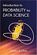Introduction to Probability for Data Science (Stanley Chan)

This book is an introductory textbook in undergraduate probability in the context of data science to emphasize the inseparability between data (computing) and probability (theory) in our time, with examples in both MATLAB and Python.

•Introduction to Probability, Statistics, and Random Processes

This book introduces students to probability, statistics, and stochastic processes. It provides a clear and intuitive approach to these topics while maintaining mathematical accuracy. The book contains a large number of solved exercises.

•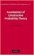Foundations of Constructive Probability Theory (Yuen-Kwok Chan)

This book provides a systematic and general theory of probability within the framework of Constructive Mathematics. It can serve as a parallel introduction into constructive mathematics and rigorous probability theory.

•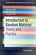Introduction to Random Matrices: Theory and Practice

This is a book for absolute beginners. The aim is to provide a truly accessible introductory account of Random Matrix theory. Most chapters are accompanied by MATLAB codes to guide readers through the numerical check of most analytical results.

•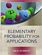Elementary Probability for Applications (Rick Durrett)

This clear and lively introduction to probability theory concentrates on the results that are the most useful for applications, including combinatorial probability and Markov chains. Concise and focused, it is designed for students familiar with basic calculus.

•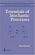Essentials of Stochastic Processes (Rick Durrett)

Stochastic processes have become important for many fields, including mathematical finance and engineering. Written by one of the worlds leading probabilists, this book presents recent results previously available only in specialized monographs.

•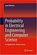Probability in Electrical Engineering and Computer Science

This textbook motivates and illustrates the techniques of applied probability by applications in electrical engineering and computer science (EECS). Python labs enable the readers to experiment and consolidate their understanding.

•Introduction to Probability (Charles M. Grinstead, et al)

The book is a beautiful introduction to probability theory at the beginning level. The book contains a lot of examples and an easy development of theory without any sacrifice of rigor, keeping the abstraction to a minimal level.

•Probability Theory: The Logic of Science (E. T. Jaynes)

Going beyond the conventional mathematics of probability theory, this study views the subject in a wider context. It discusses new results, along with applications of probability theory to a variety of problems.

•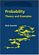Probability: Theory and Examples (Rick Durrett)

This lively introduction to measure-theoretic probability theory covers laws of large numbers, central limit theorems, random walks, martingales, Markov chains, ergodic theorems, and Brownian motion.

•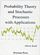Probability Theory and Stochastic Processes with Applications

This book provides an introduction to probability theory and discrete and continuous stochastic processes and its applications. It has a unique approach that provides a broad and wide introduction into the fascinating area of probability theory.

•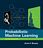Probabilistic Machine Learning: An Introduction (Kevin Murphy)

This book is a comprehensive introduction to machine learning that uses probabilistic models and inference as a unifying approach. It is written in an informal, accessible style, complete with pseudo-code for the most important algorithms.

Book Categories
 :All CategoriesRecent BooksMiscellaneous BooksComputer LanguagesComputer ScienceData Science/DatabasesElectrical EngineeringJava and Java EE (J2EE)Linux and UnixMathematicsMicrosoft and .NETMobile ComputingNetworking and CommunicationsSoftware EngineeringSpecial TopicsWeb Programming
Other Categories